Скачать презентацию Equity Valuation b Basic Types of Models

807b9d679b7547410d9dee67f6aeb744.ppt

• Количество слайдов: 18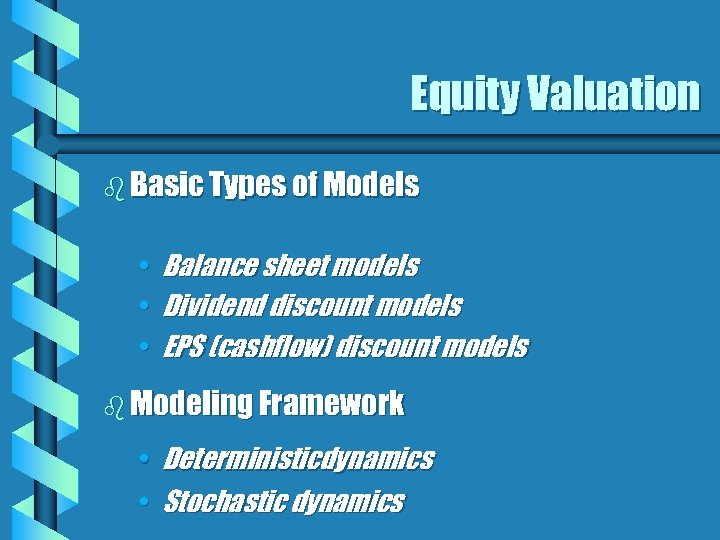Equity Valuation b Basic Types of Models • • • Balance sheet models Dividend discount models EPS (cashflow) discount models b Modeling Framework • Deterministicdynamics • Stochastic dynamics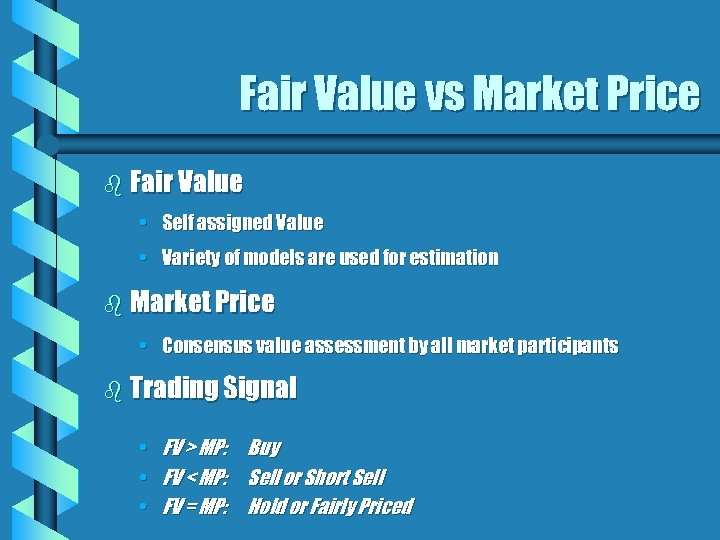Fair Value vs Market Price b Fair Value • Self assigned Value • Variety of models are used for estimation b Market Price • Consensus value assessment by all market participants b Trading Signal • FV > MP: Buy • FV < MP: Sell or Short Sell • FV = MP: Hold or Fairly Priced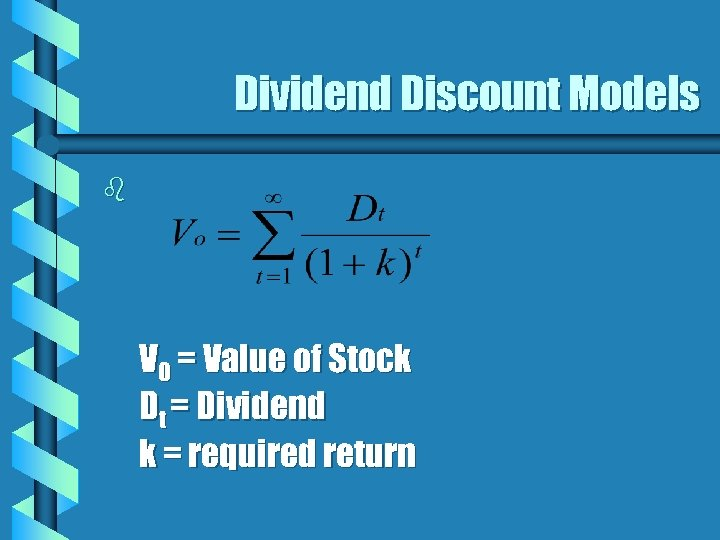Dividend Discount Models b V 0 = Value of Stock Dt = Dividend k = required return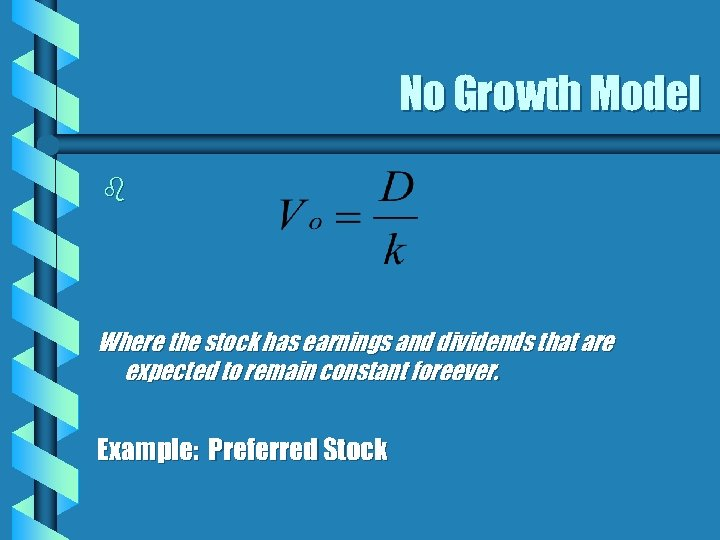No Growth Model b Where the stock has earnings and dividends that are expected to remain constant foreever. Example: Preferred Stock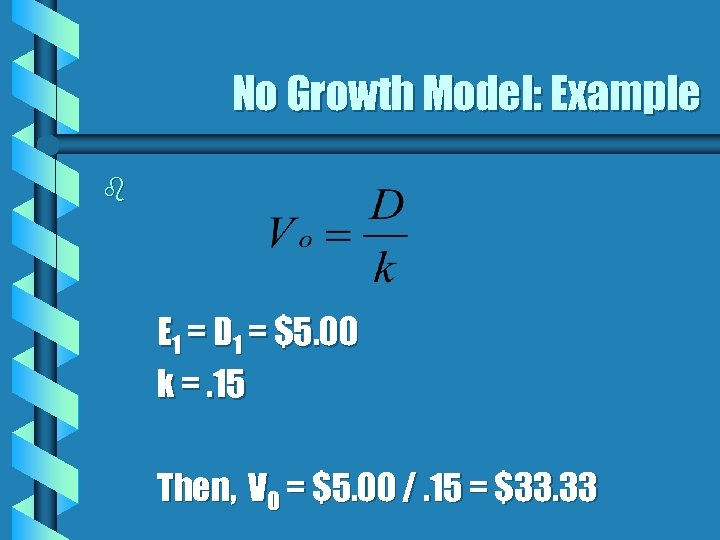No Growth Model: Example b E 1 = D 1 = \$5. 00 k =. 15 Then, V 0 = \$5. 00 /. 15 = \$33. 33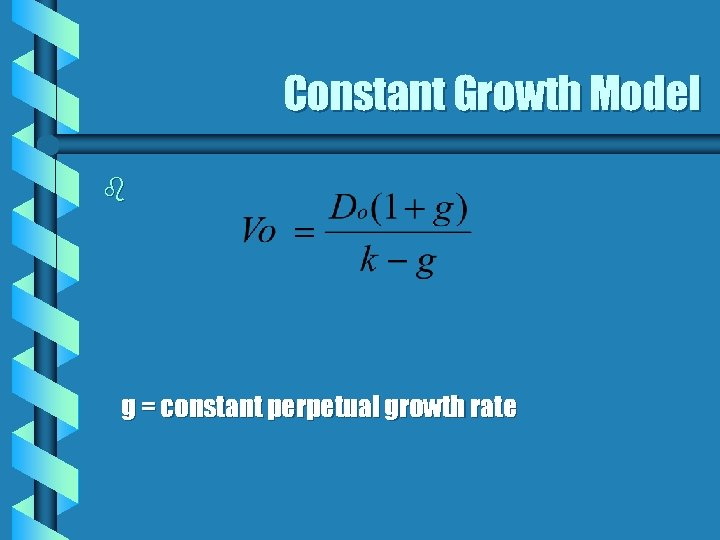Constant Growth Model b g = constant perpetual growth rate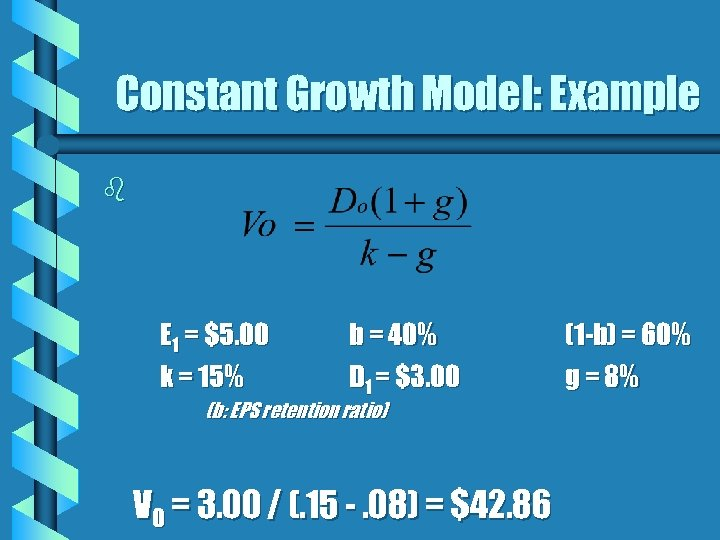Constant Growth Model: Example b E 1 = \$5. 00 k = 15% b = 40% D 1 = \$3. 00 (b: EPS retention ratio) V 0 = 3. 00 / (. 15 -. 08) = \$42. 86 (1 -b) = 60% g = 8%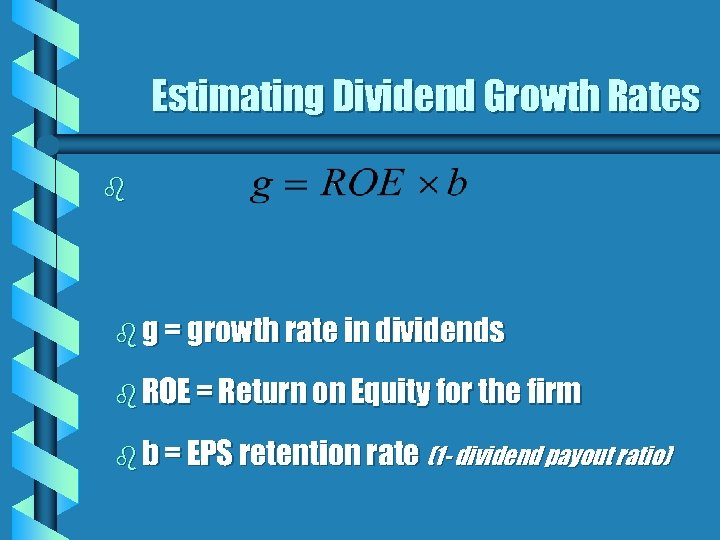Estimating Dividend Growth Rates b b g = growth rate in dividends b ROE = Return on Equity for the firm b b = EPS retention rate (1 - dividend payout ratio)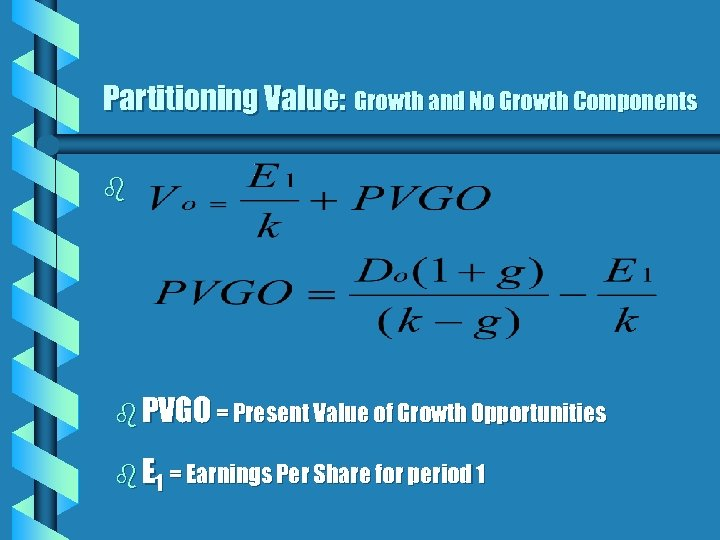Partitioning Value: Growth and No Growth Components b b PVGO = Present Value of Growth Opportunities b E 1 = Earnings Per Share for period 1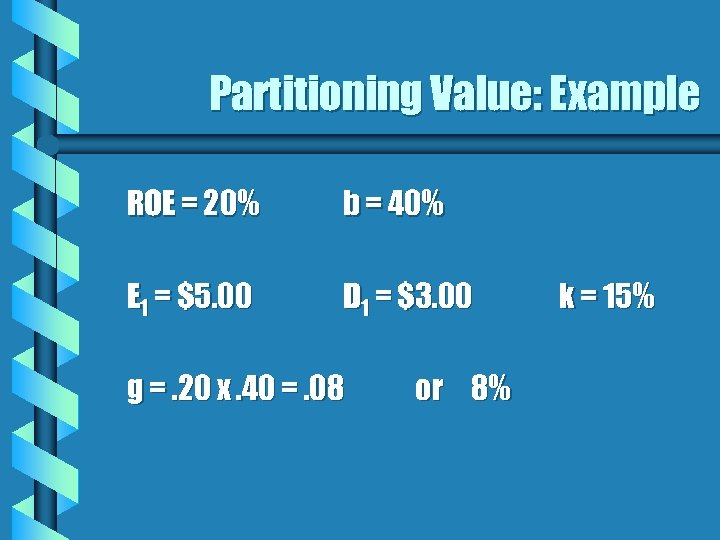Partitioning Value: Example ROE = 20% b = 40% E 1 = \$5. 00 D 1 = \$3. 00 g =. 20 x. 40 =. 08 or 8% k = 15%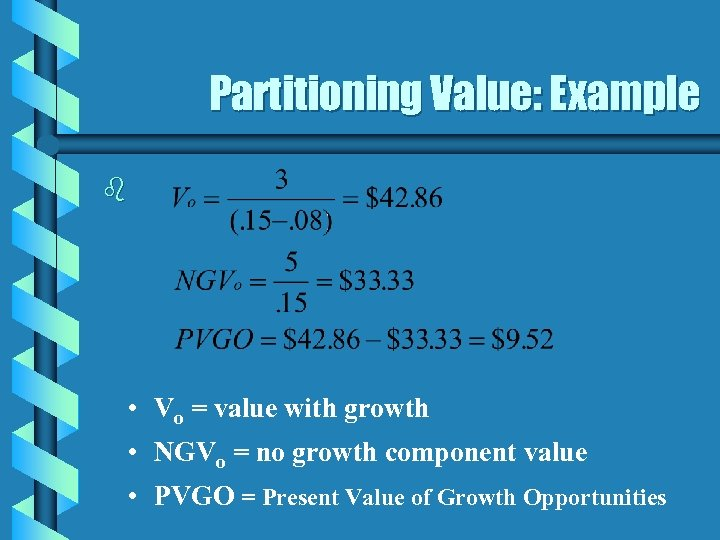Partitioning Value: Example b • Vo = value with growth • NGVo = no growth component value • PVGO = Present Value of Growth Opportunities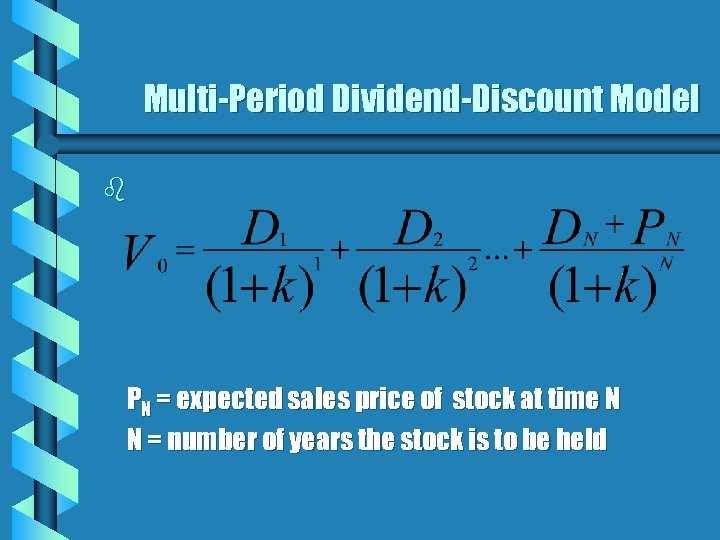Multi-Period Dividend-Discount Model b PN = expected sales price of stock at time N N = number of years the stock is to be held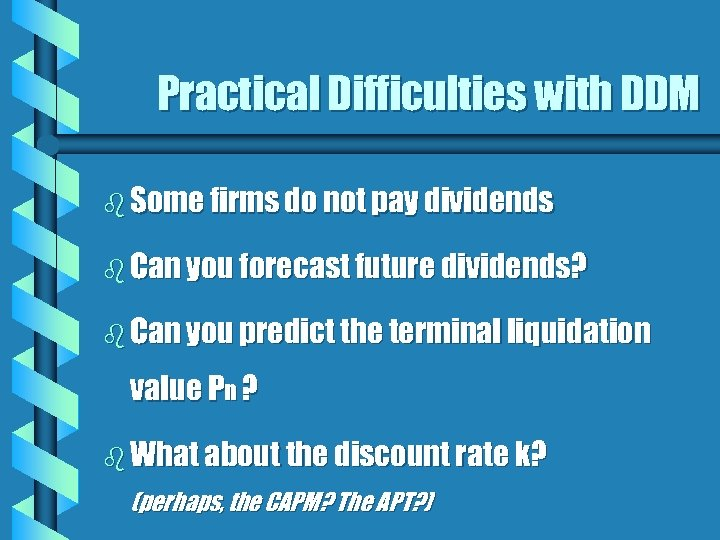Practical Difficulties with DDM b Some firms do not pay dividends b Can you forecast future dividends? b Can you predict the terminal liquidation value Pn ? b What about the discount rate k? (perhaps, the CAPM? The APT? )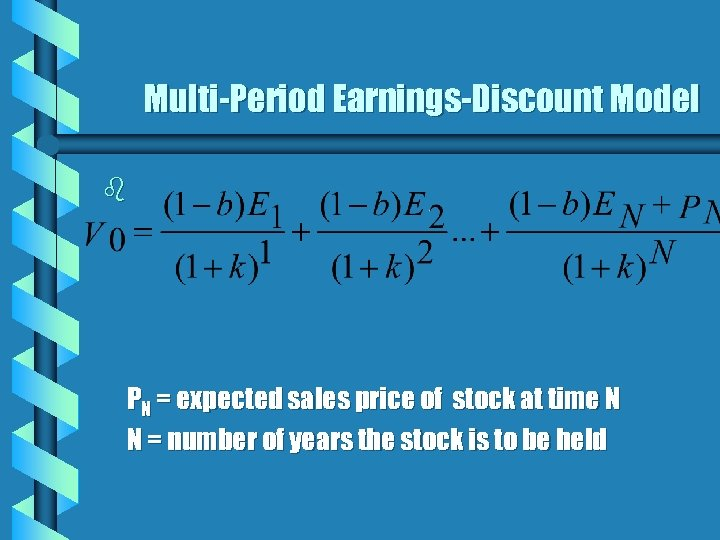Multi-Period Earnings-Discount Model b PN = expected sales price of stock at time N N = number of years the stock is to be held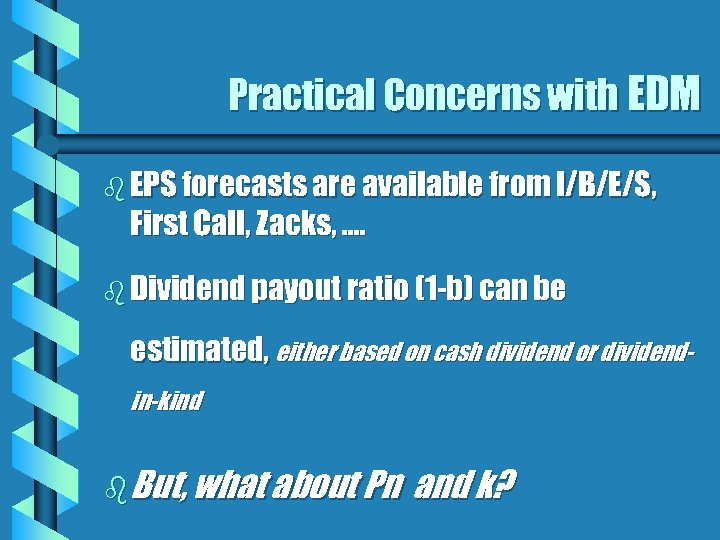Practical Concerns with EDM b EPS forecasts are available from I/B/E/S, First Call, Zacks, …. b Dividend payout ratio (1 -b) can be estimated, either based on cash dividend or dividendin-kind b But, what about Pn and k?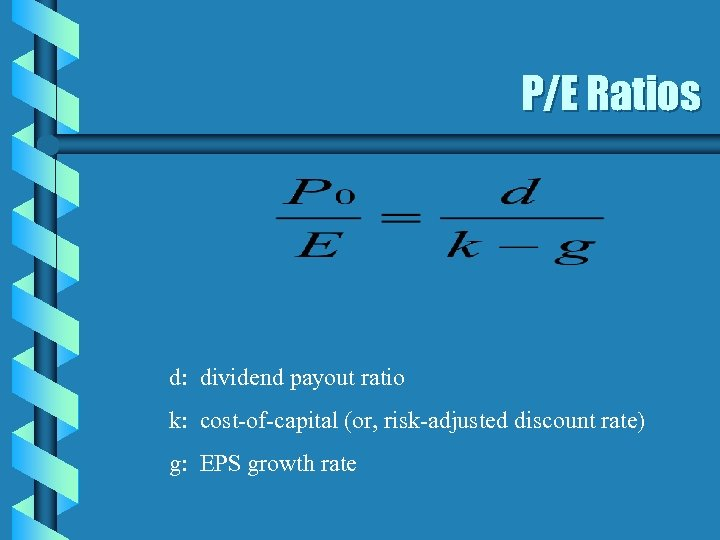P/E Ratios d: dividend payout ratio k: cost-of-capital (or, risk-adjusted discount rate) g: EPS growth rate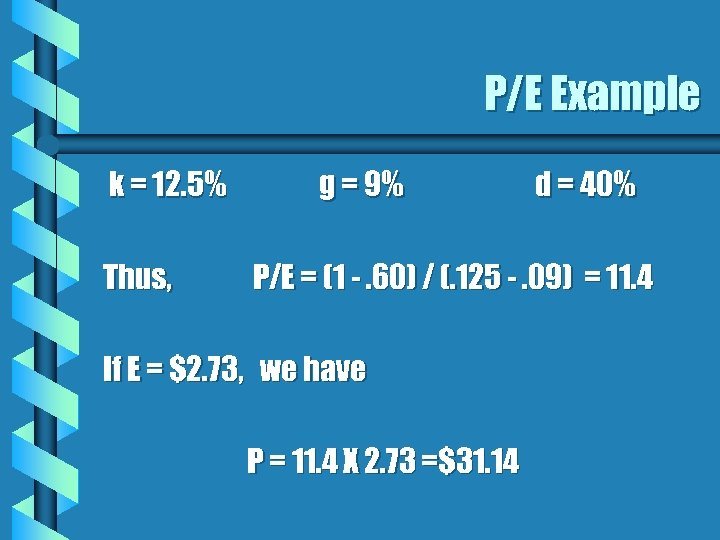P/E Example k = 12. 5% Thus, g = 9% d = 40% P/E = (1 -. 60) / (. 125 -. 09) = 11. 4 If E = \$2. 73, we have P = 11. 4 X 2. 73 =\$31. 14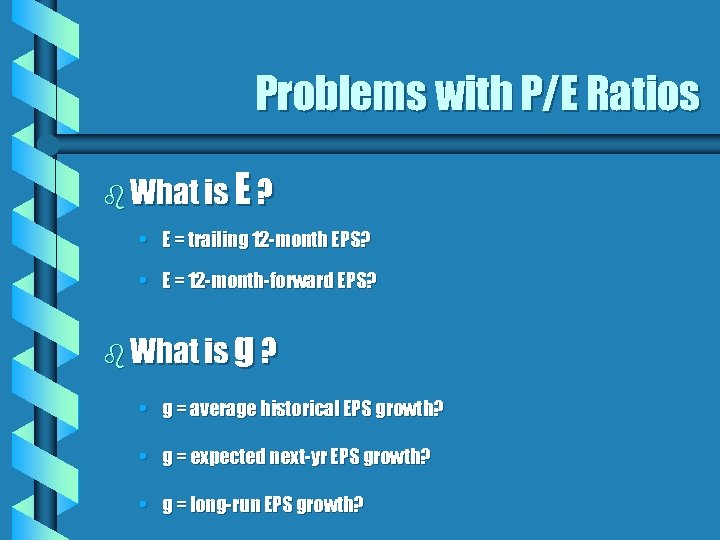Problems with P/E Ratios b What is E? • E = trailing 12 -month EPS? • E = 12 -month-forward EPS? b What is g? • g = average historical EPS growth? • g = expected next-yr EPS growth? • g = long-run EPS growth?